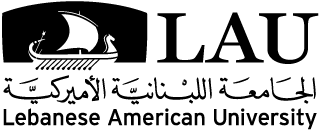# Master of Science in Applied and Computational Mathematics

## Mission

The mission of the M.S. in Applied and Computational Mathematics program is to provide a high quality teaching, research, and service to the university community, to Lebanon and the region, in the application of mathematics to other disciplines. It seeks to educate students about interdisciplinary problems, to connect them with a broad range of sciences, and to engage them in problems motivated by other sciences.

## Program Objectives

Through the M.S. in Applied and Computational Mathematics students will:

1. be exposed to the interactions between applied mathematics and other disciplines;
2. acquire specialized knowledge in the areas of numerical analysis, scientific computing, optimization, and variational analysis;
3. gain the necessary skills and tools to mathematically model and solve real-life related problems, both analytically and computationally;
4. be provided with the appropriate mathematical background for students who wish to pursue PhD studies in applied mathematics or other related fields.

## Learning Outcomes

Upon completing the program, students shall be able to:

1. develop and demonstrate the ability to reason mathematically by constructing mathematical proofs, modeling and simulating real life problems;
2. make conjectures and form hypotheses, test the accuracy of their work, and effectively solve problems analytically or numerically;
3. identify fundamental concepts of mathematics as applied to the sciences;
4. communicate mathematical ideas effectively in written and oral form;
5. acquire important techniques in optimization and control theory;
6. learn how the newly developed non-smooth analysis theory surfaces in optimization and control theory;
7. apply the learned techniques, such as optimality conditions and algorithms, construction of smooth and nonsmooth feedback controls and solutions to Hamilton-Jacobi equations, to problems stemming from engineering, economics, etc.;
8. select, develop, and apply appropriate numerical methods to estimate the solution of mathematical problems and to simulate applied problems arising in the sciences and engineering;
9. employ efficient and accurate programming and computing tools to solve applied problems.

Applicants should hold a B.S. degree in mathematics, computer science, physics, engineering, or other related fields.

Non-math majors may have to take some undergraduate mathematics remedial courses in order to join the M.S. program. The remedial courses are prescribed on a case-by-case basis; however, a minimal knowledge in real, differential, and functional analysis is expected.

## Curriculum

Graduation requires 30 credits, distributed as follows:

• Thesis (6 credits)

Core Courses

• ACM710 Scientific Computing (3 cr.)
• ACM720 Convex Analysis and Optimization (3 cr.)

Elective Courses

• ACM701 Measure Theory and Functional Analysis (3 cr.)
• ACM702 Ordinary and Partial Differential Equations (3 cr.)
• ACM711 Numerical Methods for Partial Differential Equations (3 cr.)
• ACM712 Finite Element Methods (3 cr.)
• ACM713 Numerical Linear Algebra (3 cr.)
• ACM714 Parallel Programming (3 cr.)
• ACM715 Mathematical Biology (3 cr.)
• ACM721 Nonsmooth Analysis and Applications (3 cr.)
• ACM722 Nonsmooth Optimal Control (3 cr.)
• ACM723 Calculus of Variations (3 cr.)
• ACM724 Linear Programming (3 cr.)
• ACM725 Non-Linear Programming (3 cr.)
• ACM726 Integer Programming (3 cr.)
• ACM727 Mathematical Methods for Applied Economics (3 cr.)
• ACM788 Advanced Topics in Applied and Computational Mathematics (3 cr.)